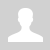21:41
Siemens S7 1200 Modbus RTU Inovance MD200 Tutorial by plc247.com

The PLC model I use is Siemens S7-1200 combined with Module S-1200 CB1241 (can also use CM1241) to connect to RS485 port of Inovance MD200.

#### PLC Siemens & Inovance MD200 “Wiring Diagram”

Below is the RS485 communication connection diagram between PLC S7-1200 and Inovance MD200.

#### Inovance MD200 Modbus Configuration

In addition to the basic parameters of the inverter installed according to the motor (frequency, voltage, current …) in order for the inverter to be controlled and monitored by Modbus RTU, we need to install additional following parameters

##### Note: Siemens S7-1200 Holding Registers Address = “VFD Address (Dec) + 40001“

>>> VFD Control Address = 2000 (hex) = 8192 (dec) + 40001 = 48193 (dec)

• Set Word 48193 = 1 >>> Forward Run
• Set Word 48193 = 2 >>> Reverse Run
• Set Word 48193 = 6 >>> Stop

>>> Set Frequency Address = 1000 (hex) = 4096 (dec) + 40001 = 44097 (dec)
>>> Frequency Reference = (0 to 10000 dec) ~ (0 to VFD Max Frequency)

• Output Frequency Address = 1001 (hex) = 4097 (dec) + 40001 = 44098 (dec)
• Output Voltage Address = 1003 (hex) = 4099 (dec) + 40001 = 44100 (dec)
• Output Current Address = 1004 (hex) = 4100 (dec) + 40001 = 44101 (dec)

#### PLC Siemens “Modbus RTU” Configuration

We need to set the communication parameters for the PLC similar to those of the Inovance MD200

+ Create Data types

+ Modbus Block Create

### PLC Siemens Modbus Example

+ Communication Configuration

+ Frequency Setting

+ VFD Control Command